# Area of Parallelogram Worksheet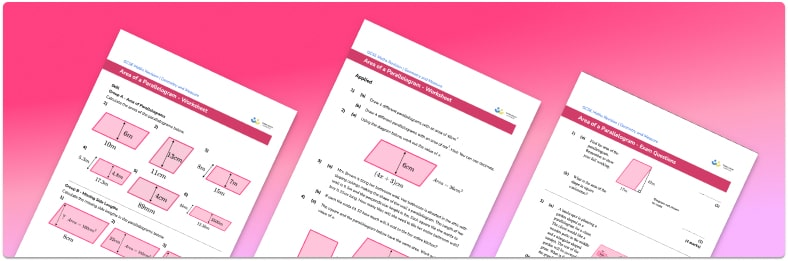• Section 1 of the printable area of parallelogram worksheet contains 15 skills-based area of a parallelogram questions, in 3 groups to support differentiation
• Section 2 contains 4 applied area of a parallelogram questions with a mix of worded problems and deeper word problem solving questions
• Section 3 contains 4 foundation and higher level GCSE exam style questions
• Answers and a mark scheme for all questions are provided
• Questions follow variation theory with plenty of opportunities for students to work independently at their own level
• All questions created by fully qualified expert secondary maths teachers
• Suitable for GCSE maths revision for AQA, OCR and Edexcel exam boards
• Other free printable worksheets with answer keys are available for related topics, such as area of a triangle, area of a trapezium and area of a circle

• This field is for validation purposes and should be left unchanged.

You can unsubscribe at any time (each email we send will contain an easy way to unsubscribe). To find out more about how we use your data, see our privacy policy.

### Area of parallelogram at a glance

Area is the amount of space covered by a 2D shape and is measured in square units – metric units of area include mm², cm² and m². At GCSE, students are expected to find the area of a few different quadrilaterals, including the area of trapezoids, parallelograms and rhombuses.

The formula for the area of a parallelogram is similar to the formula for the area of a rectangle. To work out the area of a parallelogram, we use the formula base perpendicular height. As a rhombus is a parallelogram with four equal sides, this formula also works to find the area of a rhombus.

Sometimes students are given the total area of the parallelogram, and are asked to find the height of the parallelogram or the length of the base. To do this, divide the area by the given side length. This can require students to work with decimals as well as whole numbers so it is important these skills are secure. They may also need to convert between different metric units – for example, if one side is given in centimetres and the other in millimetres.

In addition to using integers for the sides of the parallelogram, decimals and fractions are also common – for example, applying multiplication of fractions to the area of a parallelogram.

Looking forward, students can then progress to additional geometry worksheets, for example, a 3D shapes worksheet or angles in polygons worksheet.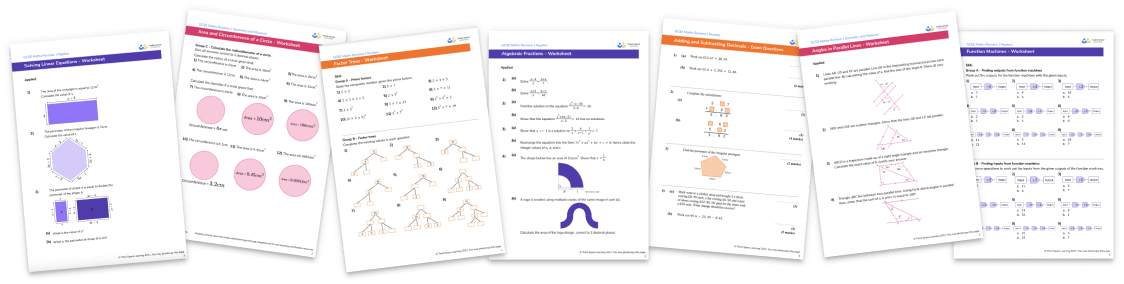For more teaching and learning support on Geometry our GCSE maths lessons provide step by step support for all GCSE maths concepts.

## Related worksheets

Area of a Circle Worksheet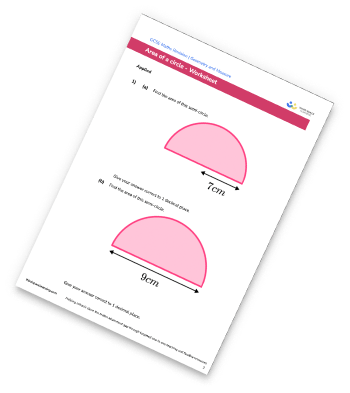Area of Compound Shapes Worksheet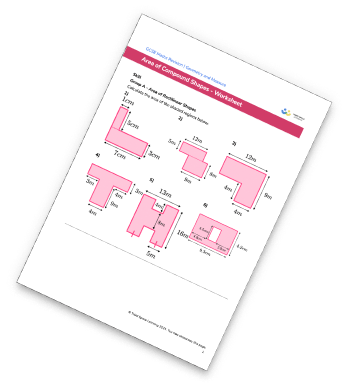Area Of A Trapezium Worksheet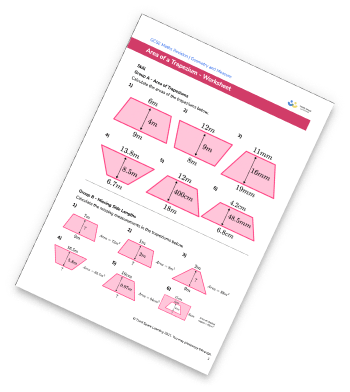Area Of A Rhombus Worksheet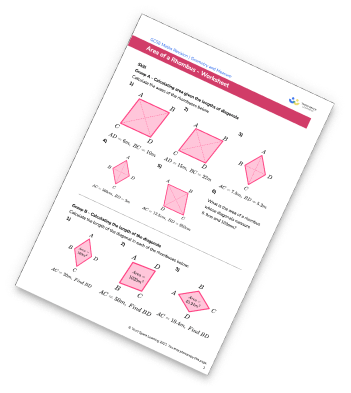## Do you have KS4 students who need more focused attention to succeed at GCSE?There will be students in your class who require individual attention to help them succeed in their maths GCSEs. In a class of 30, it’s not always easy to provide.

Help your students feel confident with exam-style questions and the strategies they’ll need to answer them correctly with our dedicated GCSE maths revision programme.

Lessons are selected to provide support where each student needs it most, and specially-trained GCSE maths tutors adapt the pitch and pace of each lesson. This ensures a personalised revision programme that raises grades and boosts confidence.

Find out more